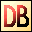DelphiBasicsLnFunction Gives the natural logarithm of a number System unit
 function Ln ( Number : Extended ) : Extended;
Description
The Ln function takes an integer or floating point Number and gives the natural logarithm of that number.

This is only used for mathematical processing.

Ln has the opposite effect to Exp - the exponent of a number. (2.72 raised to that number power).
Related commands
 Exp Gives the exponent of a number Log10 Gives the log to base 10 of a numberDownload this web site as a Windows program.